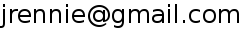# SMART Word Vector Weightings

Below is a description of the word weighting schemes found in the SMART source code (src/libconvert/weight_tri.c). A weighting is described by the three letters corresponding to the tf, idf and normalization steps, such as "atc".

```  The value of the parameter is a 3 character code (eg "atc"):
First char gives the term-freq procedure to be used
Second char gives the inverted-doc-freq procedure to be used.
Third char gives the normalization procedure to be used.

There are three possible conversion that can be performed on each vector:
1. Normalize term-freq component - most often the tf component is
altered by dividing by the max tf in the vector
2. Alter the doc weight, possibly based on collection freq info.
Note that this is done individually on each term
3. Normalize the entire subvector - most often to "sum of squares" of
terms = 1. Alternative is sum of terms = 1

Weighting schemes and the desired weight-type parameter
Parameters are specified by the first character of the incoming string
1.  "none"     : new-tf = tf
No conversion to be done  1 <= new-tf
"binary"   : new-tf = 1
"max-norm" : new-tf = tf / max-tf
divide each term by max in vector  0 < new-tf < 1.0
"aug-norm" : new-tf = 0.5 + 0.5 * (tf / max-tf)
augmented normalized tf.  0.5 < new-tf <= 1.0
"square"   : new-tf = tf * tf
"log"      : new-tf = ln (tf) + 1.0

2.  "none"     : new-wt = new-tf
No conversion is to be done
"tfidf"    : new-wt = new-tf * log (num-docs/coll-freq-of-term)
Usual tfidf weight (Note: Pure idf if new-tf = 1)
"prob"     : new-wt = new-tf * log ((num-docs - coll-freq)
/ coll-freq))
Straight probabilistic weighting scheme
"freq"     : new-wt = new-tf / n
"squared"  : new-wt = new-tf * log(num-docs/coll-freq-of-term)**2

3.  "none"     : norm-weight = new-wt
No normalization done
"sum"      : divide each new-wt by sum of new-wts in vector
"cosine"   : divide each new-wt by sqrt (sum of (new-wts squared))
This is the usual cosine normalization (I.e. an
inner product function of two cosine normalized
vectors will yield the same results as a cosine
function on vectors (either normalized or not))
"fourth"   : divide each new-wt by sum of (new-wts ** 4)
"max"      : divide each new-wt by max new-wt in vector
```

SMART is available via FTP from Cornell (smart.11.0.tar.Z)